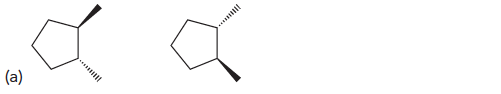# Problem: For each of the following pairs of compounds, determine the relationship between the two compounds:

🤓 Based on our data, we think this question is relevant for Professor Wetzler's class at CLEMSON.

###### Problem Details

For each of the following pairs of compounds, determine the relationship between the two compounds:What scientific concept do you need to know in order to solve this problem?

Our tutors have indicated that to solve this problem you will need to apply the What is the Relationship Between Isomers? concept. You can view video lessons to learn What is the Relationship Between Isomers?. Or if you need more What is the Relationship Between Isomers? practice, you can also practice What is the Relationship Between Isomers? practice problems.

What is the difficulty of this problem?

Our tutors rated the difficulty ofFor each of the following pairs of compounds, determine the ...as low difficulty.

What professor is this problem relevant for?

Based on our data, we think this problem is relevant for Professor Wetzler's class at CLEMSON.

What textbook is this problem found in?

Our data indicates that this problem or a close variation was asked in Organic Chemistry - Klein 1st Edition. You can also practice Organic Chemistry - Klein 1st Edition practice problems.